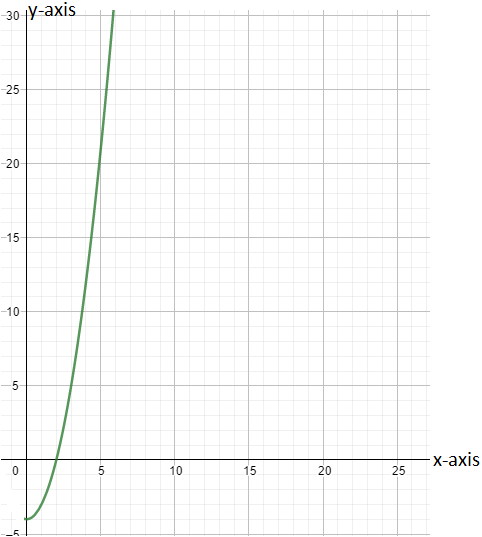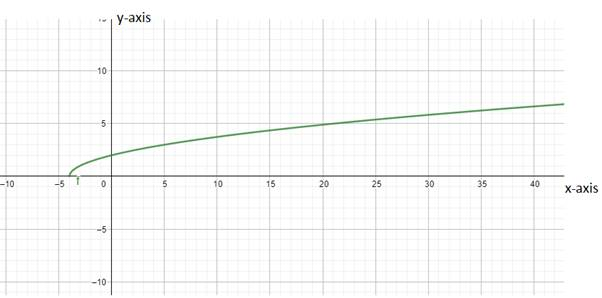# To draw: Graph of the function### Precalculus: Mathematics for Calcu...

6th Edition
Stewart + 5 others
Publisher: Cengage Learning
ISBN: 9780840068071### Precalculus: Mathematics for Calcu...

6th Edition
Stewart + 5 others
Publisher: Cengage Learning
ISBN: 9780840068071

#### Solutions

Chapter 2, Problem 91RE

a.

To determine

## To draw: Graph of the function

Expert Solution

### Explanation of Solution

Given information:

f(x)=x24    x0

Graph : To draw the graph of f1 if the f(x) is given then switch all the x and y values in each point to graph the inverse. Just look at those values switching places from the f(x) function to its inverse g(x) reflected over the line y=xInterpretation :

The values switching places from the f(x) function to its inverse g(x) are reflected over the line y=x

b.

To determine

Expert Solution

### Explanation of Solution

Given information:

f(x)=x24    x0

Graph: To draw the graph of f1 if the f(x) is given then switch all the x and y values in each point to graph the inverse. Following is the graph of f1 .Interpretation :

The values switching places from the f(x) function to its inverse g(x) reflected over the line y=x

c.

To determine

Expert Solution

f1(x)=x+4

### Explanation of Solution

Given information:

f(x)=x24    x0

Calculation:

To find the equation for f1

y=f(x)y=x24

Swap ‘x’ with ‘y’

x=y24

y2=x+4y=x+4   f1(x)=x+4

is the required equation.

After swapping the values of x and y, solve the equation for y by bringing like terms together and the final value of y is the inverse of the function i.e. f1(x) .

### Have a homework question?

Subscribe to bartleby learn! Ask subject matter experts 30 homework questions each month. Plus, you’ll have access to millions of step-by-step textbook answers!